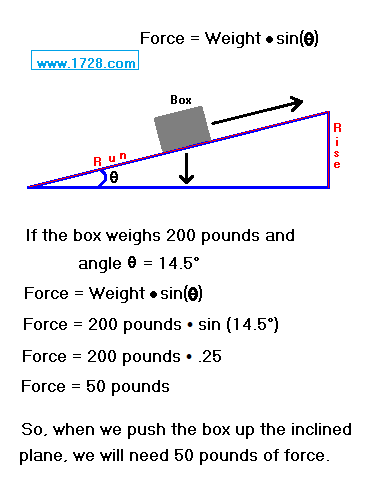6 Simple Machines

 The Inclined PlaneOf all the six simple machines, the inclined plane is the simplest, having no moving parts and was probably the first to be "discovered". An inclined plane's mechanical advantage is always greater than one. Let's suppose we measure the height of the plane and find it is 3 inches for every foot of length. For every foot we wish to raise the box (the rise), we have to push it four feet horizontally (the run), giving us a mechanical advantage of 4. The force required to move an object up an inclined plane equals its weight times the sine of the inclined plane's angle. Force = weight • sine (q) If we are not given the angle, we can still calculate it. We know the "rise" is 1 and the "run" is 4, so Angle (q) = arc sine (.25)   Angle (q) = 14.5° Return To Home Page Copyright © 1999 -     1728 Software Systems